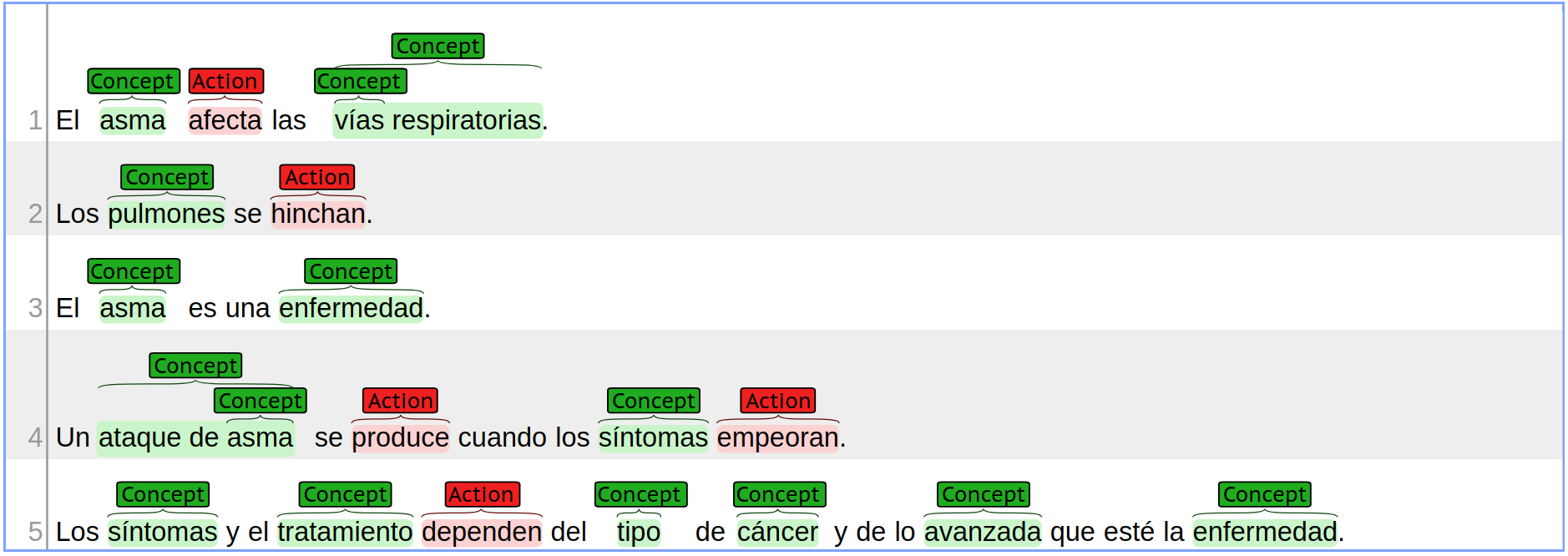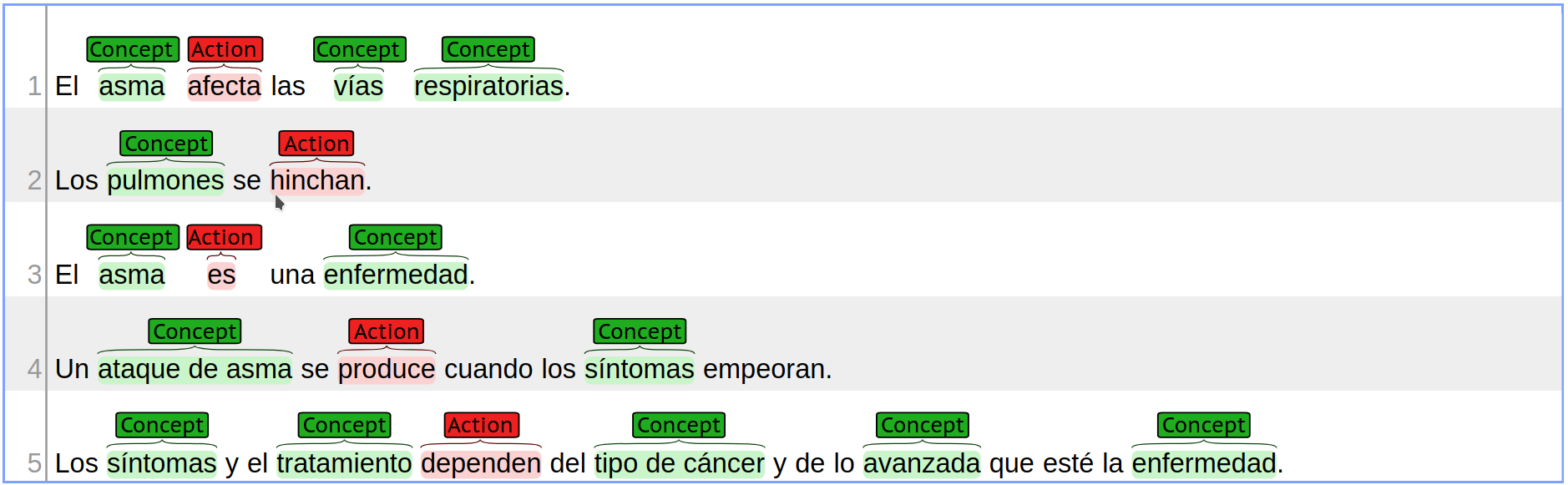# TASS-2018-Task 3. eHealth Knowledge Discovery

## Subtask B: Classification of key phrases

Subtask B continues where Subtask A ends. Given an input file in plain text and the corresponding output from Subtask A, where the text spans that appear in the input have been identified, the purpose of Subtask B is to assign a label to each of these text spans. The labels considered have been inspired by the research in Teleologies , and can be Concept , or Action . The dev output file created per document is named output_B_<topic>.txt . For example output_B_example.txt .

The corresponding gold labels for the example document presented in the previous section are illustrated in the following figure. The corresponding output file is presented below.

#### Example gold output illustration:

This illustration represents the following gold files:#### Example gold output file:

A brief description of each label follows:

• Concept: the concepts are those key phrases that are able to represent objects and other entities presumed to be of interest for some particular purpose. It is possible to represent simple and complex concepts. Simple concepts just represent singular entries like “asma” or multi-words like “vías respiratorias”, etc. Complex concepts are out scope for this Subtask B because they consist of a group of different concepts.
• Action : is a type of Concept which provokes a modification of another Concept , commonly represented by verbs or phrases that include verbs, but in some cases it can be represented by a non-verb. This Subtask only requires the recognition of actions and it is not necessary to detect who performs the action . That problem is tackled in Subtask C.

For each keyphrase identified in Subtask A one of the aforementioned two labels is given. The format is one entry per line, consisting of a number and a label separated by a single whitespace (or tab). The number matches the ID of one of the text spans identified in Subtask A. It is not necessary for the entries to appear in order, since the matching between a LABEL and the corresponding text is done by the ID field.

## Development evaluation of Subtask B

When the evaluation process is carried out the gold and dev files do not need to assign the same ID to the same text spans. When processing the Subtask A, the evaluator builds a mapping between the corresponding IDs in each file, where matching Correct or Partial items are mapped together. The LABEL comparisons in Subtask B will use this mapping to find out the corresponding expected label for each text span.

The following figure and file output represent a development example output ( output_B_example.txt ) which includes some incorrect labelling of the example input:

#### Example dev output illustration:

Example dev file:#### Example dev file output:

The output is the evaluation script relevant for this Subtask is the following:

The script reports Correct , Incorrect , Missing , and Spurious labels:

• Correct are those phrases labelled with the same label in both the gold and the dev files. These are necessarily phrases which were either correctly or partially matched in Subtask A.
• Incorrect are those with different labels. Also, these are phrases which were either correctly or partially matched in Subtask A.
• Missing are the same as in Subtask A.
• Spurious are the same as in Subtask A.

The script reports two different accuracy metrics: micro-accuracy, which considers only the labels involving correct or partial matches in Subtask A; and, macro-accuracy, which considers all the elements:

$$micro = \frac{correct}{correct + incorrect}$$
$$macro = \frac{correct}{correct + incorrect + spurious + missing}$$

Hence, the micro accuracy is always greater or equal to the macro . The micro accuracy is a measure of the quality of Subtask B independent of mistakes made in Subtask A, while the macro accuracy is a measure of the quality of the whole process.

The script also reports standard precision , recall , and F1 computed as follows:

$$F_1 = 2 \cdot \frac{precision \cdot recall}{precision + recall}$$
$$precision = \frac{correct + \frac{1}{2} partial}{correct + incorrect + spurious + partial}$$
$$recall = \frac{correct + \frac{1}{2} partial}{correct + incorrect + missing + partial}$$
 NOTE: These metrics are only reported for convenience here, to be used by the participants when developing their solutions. The actual score used for ranking participants will be presented later.